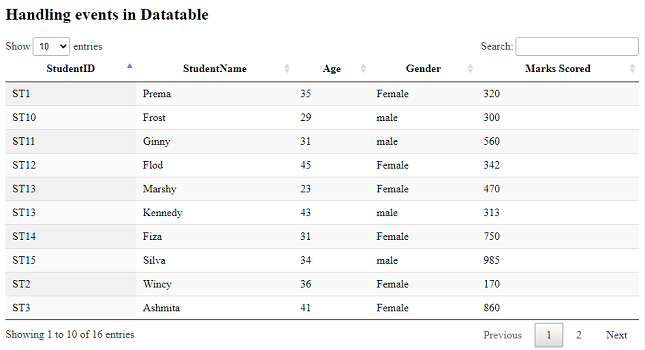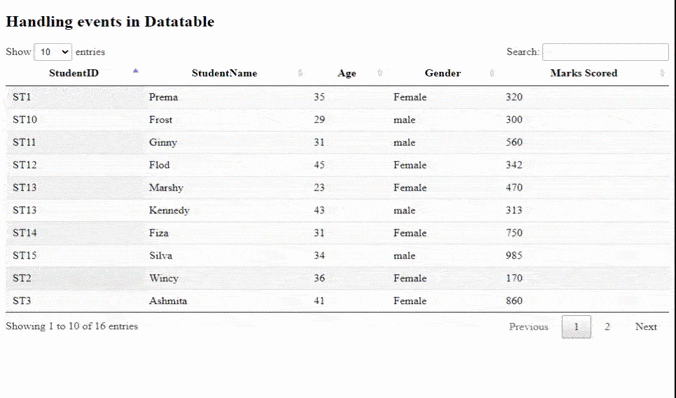# How to handle events using jQuery DataTables plugin?

• Last Updated : 18 Jan, 2021

DataTables are a modern jQuery plugin for adding interactive and advanced controls to HTML tables for our webpage. It is a simple-to-use jQuery plug-in with a huge range of many options for the developer’s custom changes. The common features of DataTables are sorting, searching, pagination, and multiple-column ordering.

In this article, we will learn to handle jQuery events using the plugin.

Hey geek! The constant emerging technologies in the world of web development always keeps the excitement for this subject through the roof. But before you tackle the big projects, we suggest you start by learning the basics. Kickstart your web development journey by learning JS concepts with our JavaScript Course. Now at it's lowest price ever!

The pre-compiled files which are needed to implement are

• CSS :

`https://cdn.datatables.net/1.10.22/css/jquery.dataTables.min.css`
• JavaScript :

`//cdn.datatables.net/1.10.22/js/jquery.dataTables.min.js`

Approach: We have an HTML table with student’s data like ID, name, age, gender, and marks scored by each of them. Another “div” with id as resultID is taken to show the result for the event triggered by the user. The plugin’s datatable is initialized with the table id in the JavaScript part of the code. The events are dealt by using jQuery’s on() method. The DataTable plugin’s row().data() method is used to get information about the selected row by the user.

Example: The following example demonstrates handling jQuery events using the DataTable plugin.

## HTML

 ```<``html``>``   ``<``head``>``      ``<``meta` `content="``initial-scale``=``1``, ``                     ``maximum-scale``=``1``, ``                     ``user-scalable``=``0``"``            ``name``=``"viewport"` `/>``      ``<``meta` `name``=``"viewport"` `            ``content``=``"width=device-width"` `/>``             ` `      ````      ``<``link` `rel``=``"stylesheet"` `            ``href``=``"https://cdn.datatables.net/1.10.22/css/jquery.dataTables.min.css"` `/>``       ` `      ````      ``<``script` `type``=``"text/javascript"` `              ``src``=``"https://code.jquery.com/jquery-3.5.1.js"``>``       ` `      ````      ``<``script` `type``=``"text/javascript"` `              ``src``=``"https://cdn.datatables.net/1.10.22/js/jquery.dataTables.min.js"``>``   ````   ``<``body``>``      ``<``h2``>Handling events in Datatable ``      ````      ``<``table` `id``=``"tableID"` `class``=``"display"` `             ``style``=``"width:100%"``>``         ``<``thead``>``            ``<``tr``>``               ``<``th``>StudentID``               ``<``th``>StudentName``               ``<``th``>Age``               ``<``th``>Gender``               ``<``th``>Marks Scored``            ````         ````         ``<``tbody``>``            ``<``tr``>``               ``<``td``>ST1``               ``<``td``>Prema``               ``<``td``>35``               ``<``td``>Female``               ``<``td``>320``            ````            ``<``tr``>``               ``<``td``>ST2``               ``<``td``>Wincy``               ``<``td``>36``               ``<``td``>Female``               ``<``td``>170``            ````            ``<``tr``>``               ``<``td``>ST3``               ``<``td``>Ashmita``               ``<``td``>41``               ``<``td``>Female``               ``<``td``>860``            ````            ``<``tr``>``               ``<``td``>ST4``               ``<``td``>Kelina``               ``<``td``>32``               ``<``td``>Female``               ``<``td``>433``            ````            ``<``tr``>``               ``<``td``>ST5``               ``<``td``>Satvik``               ``<``td``>41``               ``<``td``>male``               ``<``td``>162``            ````            ``<``tr``>``               ``<``td``>ST6``               ``<``td``>William``               ``<``td``>37``               ``<``td``>Female``               ``<``td``>372``            ````            ``<``tr``>``               ``<``td``>ST7``               ``<``td``>Chandan``               ``<``td``>31``               ``<``td``>male``               ``<``td``>375``            ````            ``<``tr``>``               ``<``td``>ST8``               ``<``td``>David``               ``<``td``>45``               ``<``td``>male``               ``<``td``>327``            ````            ``<``tr``>``               ``<``td``>ST9``               ``<``td``>Harry``               ``<``td``>29``               ``<``td``>male``               ``<``td``>205``            ````            ``<``tr``>``               ``<``td``>ST10``               ``<``td``>Frost``               ``<``td``>29``               ``<``td``>male``               ``<``td``>300``            ````            ``<``tr``>``               ``<``td``>ST11``               ``<``td``>Ginny``               ``<``td``>31``               ``<``td``>male``               ``<``td``>560``            ````            ``<``tr``>``               ``<``td``>ST12``               ``<``td``>Flod``               ``<``td``>45``               ``<``td``>Female``               ``<``td``>342``            ````            ``<``tr``>``               ``<``td``>ST13``               ``<``td``>Marshy``               ``<``td``>23``               ``<``td``>Female``               ``<``td``>470``            ````            ``<``tr``>``               ``<``td``>ST13``               ``<``td``>Kennedy``               ``<``td``>43``               ``<``td``>male``               ``<``td``>313``            ````            ``<``tr``>``               ``<``td``>ST14``               ``<``td``>Fiza``               ``<``td``>31``               ``<``td``>Female``               ``<``td``>750``            ````            ``<``tr``>``               ``<``td``>ST15``               ``<``td``>Silva``               ``<``td``>34``               ``<``td``>male``               ``<``td``>985``            ````         ````      ````      ``<``br``/>``      ``<``div` `id``=``"resultID"``>``      ``<``script``>``         ````          ``\$(document).ready(function() {               ``              ` `              ``var datatable = \$('#tableID').DataTable();``               ````               ``\$('#tableID tbody').on('click', 'tr', function () ``               ``{``                  ``var data = datatable.row( this ).data();``                  ````                  ``\$("#resultID").show().html("<``b``>User clicked on "``                  ``+data+' row '+``                  ``'<``b``> with Name: '``                  ``+data+ '<``b``> ,Age: '+data+'<``b``> ,Marks: '``                  ``+data);``             ``});``         ``});   ``              ` `      ````   `````

Output:

• Before row-click event:• After row-click event:My Personal Notes arrow_drop_up